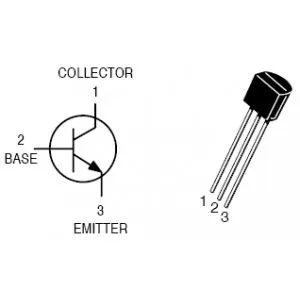# How to use BJTs © GPL3+

How to design circuits that allow you to drive a load that needs a higher value of voltage or current than Arduino can drive.

• 8,697 views
• 1 comment
• 7 respects

## Components and supplies

### 1. Why use BJTs?

When you want to drive a load that needs high values of current and/or voltage and and a digital control is needed (Arduino in this case), is not possible to use mechanical switch. For this reason electronic device that work as digital switches (ON/OFF) are used (BJT and MOS).

### 2. Electronic buttons

As for mechanical buttons the current flow can be stopped opening the positive pole or the negative one. This methods are called high-side driving or low-side respectively. This tutorial will show how to design a circuit for low-side driving.

### 3. Choose a transistor

First of all you need to choose a transistor. In this tutorial we will use the famous 2N2222, but all the rules used can be used for any NPN BJT. We will design something like this.

### 4. How does it work?

With this configuration when a `digitalWrite(pin, LOW)` instructions is used the switch is open stopping the current flow in the load, while a `digitalWrite(pin, HIGH)` instruction is used the switch is closed flowing current in the load.

### 5. What do we have to design?

Since the load (the thing that you want to turn on/off, e.g. relay, power LED) is known we can already choose the power supply value and the current the load needs to work. So we have to design only the base resistor RB.

### 6. Voltage & Current limits

The power supply value and the current that flow in the load are used to choose the transistor. In fact, with these parameters, we to check the VCEO and the Collector Current of the chosen transistor. From 2N2222 datasheet:

### 7. An example

So we have to choose a load with a power supply value <40V and a current <600mA. In this example we are going to consider a load that needs a power supply of 12V and a current of 150mA.

### 8. DC current gain

From the datasheet the DC current gain (hFE) in this conditions is 100:

### 9. How to use hfe

The hfe is necessary in order to calculate the Ib and the RB as a consequence.

``````IB=3IL/hfe=3*150mA/100 =4.5mA
``````

To design the RB we have to find in the datasheet the maximum VBEsat of the chosen transistor with our parameters (Vcc and IL).

### 10. Design the resistor base RB

In this case VBEsat = 1.2V

Now we can design the RB:

``````RB = (5V -1.2V)/4.5mA =844Ω
``````

Choosing a commercial value:

``````RB =820Ω
``````

P.S: In this example the used Arduino as a 5V logic. If we want to use a 3.3V logic (Arduino DUE):

``````RB = (3.3V -1.2V)/4.5mA =466Ω
``````

Choosing a near commercial value:

``````RB =470Ω
``````

July 8, 2016

#### Members who respect this project

See similar projects
you might like

• 5,954 views
• 1 comment
• 13 respects

#### Arduino Bluetooth Robot for Android Device

Project showcase by aip06

• 4,378 views
• 19 respects

• 13,381 views
• 11 respects

#### Arduino Bluetooth Basic Tutorial

• 661,359 views
• 390 respects

#### DHT11 /DHT22 Temperature Sensor

Project tutorial by Daniel Porrey

• 100,022 views